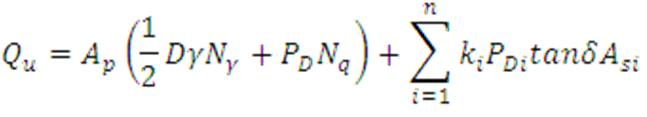# Load carrying capacity of cast in-situ piles in cohesion less soil-(IS-2911-Part1-Sec2-2010)

As per IS-2911-Part1/sec2, the ultimate load carrying capacity of pile, Qu, consists of two parts. One part is due to friction, called skin friction or shaft friction or side shear, Qs and the other is due to end bearing at the base or tip of the pile toe, Qb.The equation given below is used to calculate the ultimate load carrying capacity of pile.Where,

Ap = cross-sectional area of pile base, m2

D = diameter of pile shaft, m

γ = effective unit weight of the soil at pile tip, kN/m3

Nγ= bearing capacity factor

Nq = bearing capacity factor

Φ = Angle of internal friction at pile tip

PD = Effective overburden pressure at pile tip, in kN/m2

Ki = Coefficient of earth pressure applicable for the ith layer

PDi = Effective overburden pressure for the ith layer, in kN/m2

δi = Aangle of wall friction between pile and soil for the ith layer

Asi = Surface area of pile shaft in the ith layer, in m2

The first term is the expression for the end bearing capacity of pile (Qb) and the second term is the expression for the skin friction capacity of pile (Qs).

A minimum factor of safety of 2.5 is used to arrive at the safe pile capacity(Qsafe) from ultimate load capacity (Qu).

Qsafe = Qu/2.5

## Important Notes to remember

• The value of bearing capacity factor Nq is obtained from the figure given below.